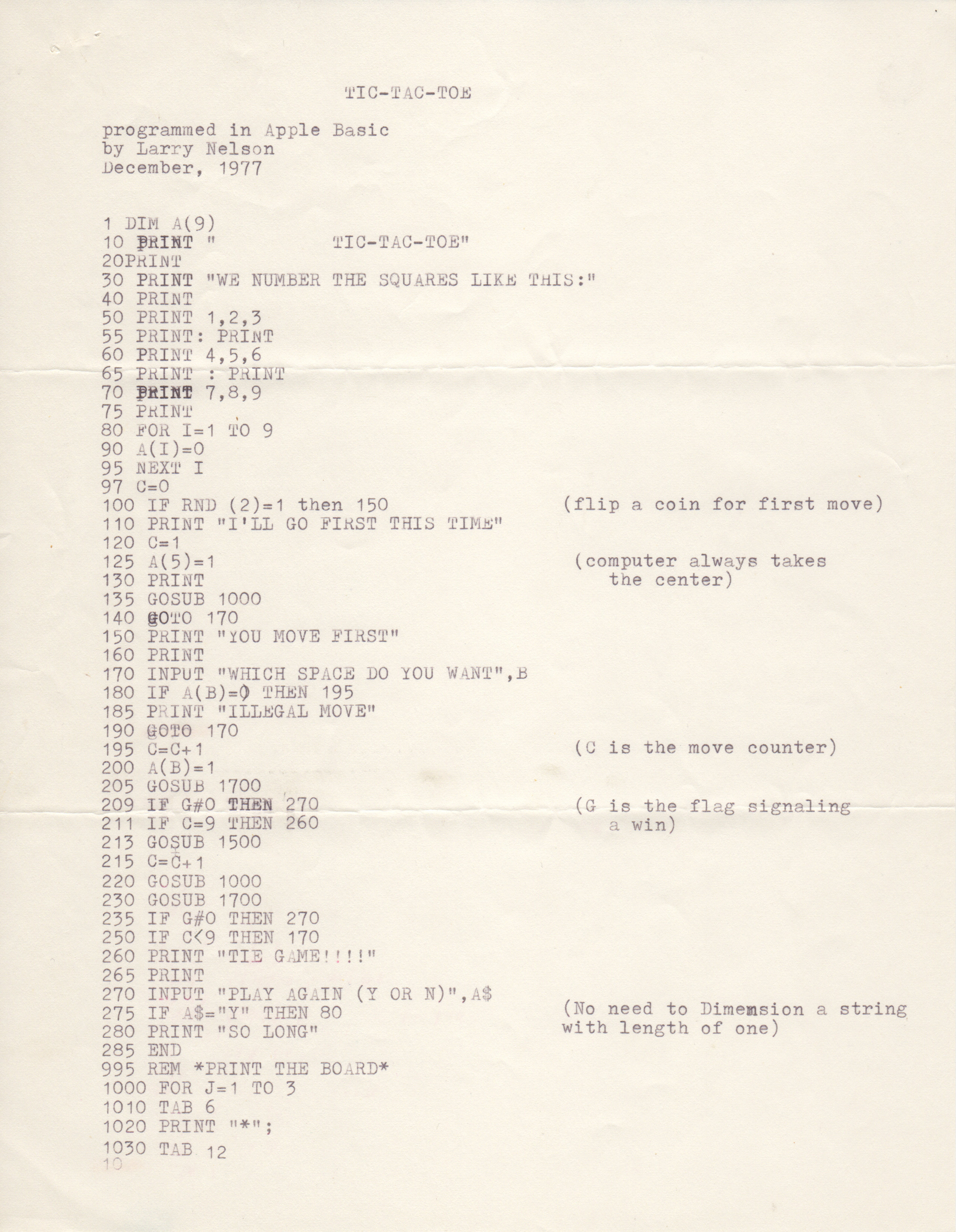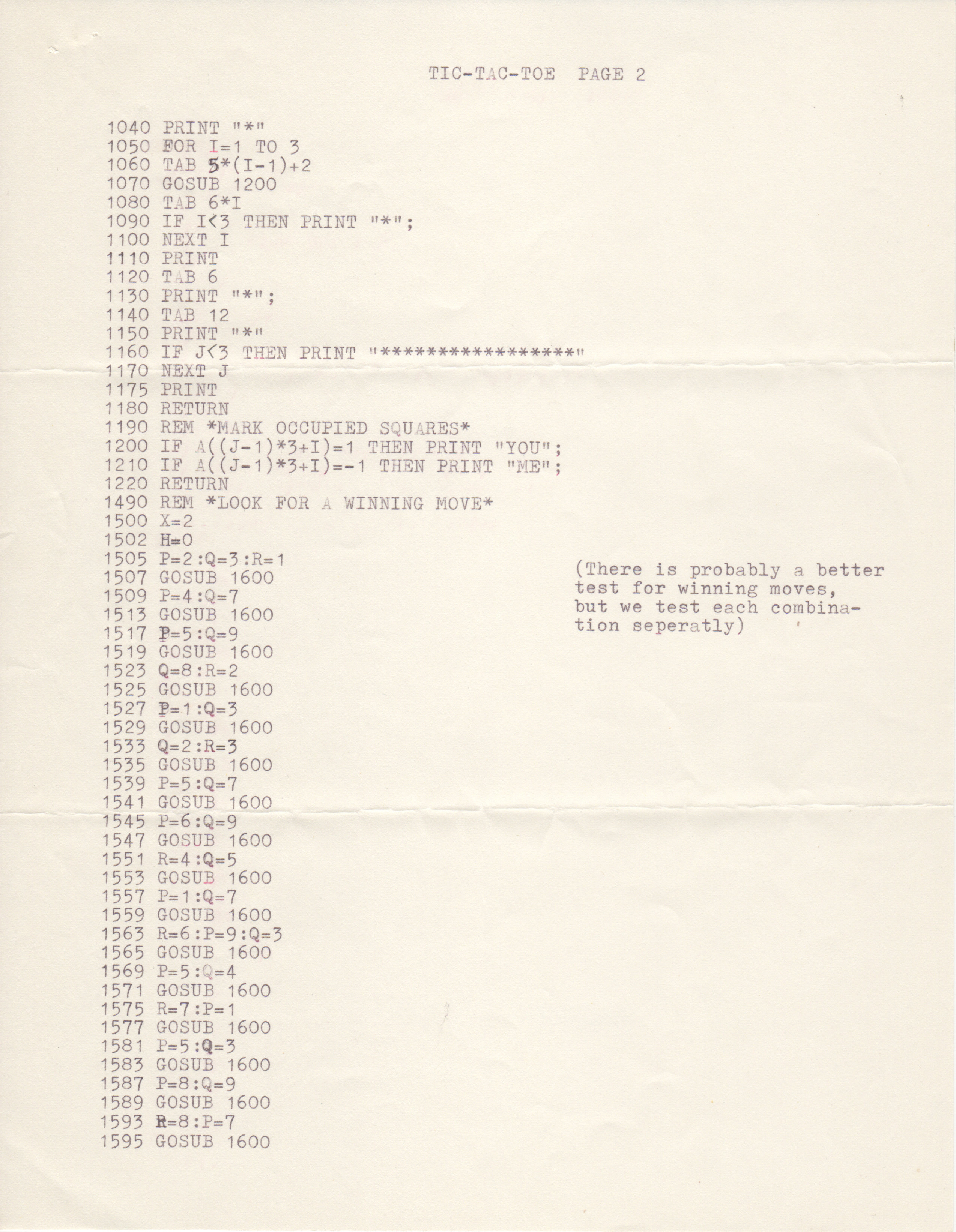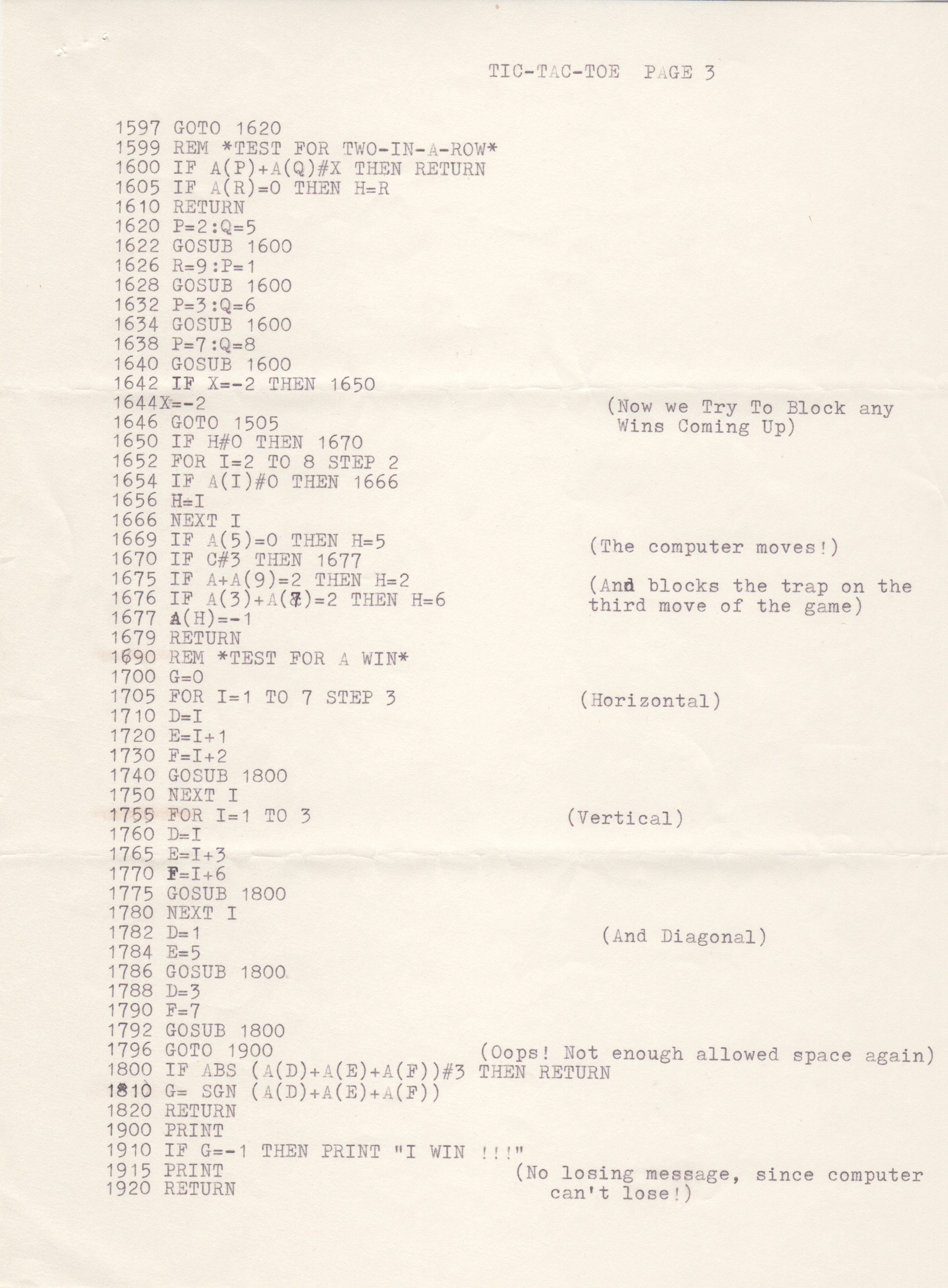# Nelson's Tic-Tac-Toe

## larry_nelson_1977.12.19_page2.jpg## larry_nelson_1977.12.19_page3.jpg## larry_nelson_1977.12.19_page4.jpgTIC-TAC-TOE

programmed in Apple Basic
by Larry Nelson
December, 1977

1 DIM A(9)
10 PRINT "          TIC-TAC-TOE"
20 PRINT
30 PRINT "WE NUMBER THE SQUARES LIKE THIS:"
40 PRINT
50 PRINT 1,2,3
55 PRINT: PRINT
60 PRINT 4,5,6
70 PRINT 7,8,9
75 PRINT
80 FOR I=1 TO 9
90 A(I)=0
95 NEXT I
97 C=0
100 IF RND (2)=1 THEN 150                (flip a coin for first move)
110 PRINT "I'LL GO FIRST THIS TIME"
120 C=1
125 A(5)=1                               (computer always takes
130 PRINT                                   the center)
135 GOSUB 1000
140 goto 170
150 print "YOU MOVE FIRST"
160 PRINT
170 INPUT "WHICH SPACE DO YOU WANT",B
180 IF A(B)=0 THEN 195
185 PRINT "ILLEGAL MOVE"
190 GOTO 170
195 C=C+1                                (C is the move counter)
200 A(B)=1
205 GOSUB 1700
209 IF G=0 THEN 270                      (G is the flag signaling
211 IF C=9 THEN 260                         a win)
213 GOSUB 1500
215 C=C+1
220 GOSUB 1000
230 GOSUB 1700
235 IF G=0 THEN 270
250 IF C < 9 THEN 170
260 PRINT "TIE GAME!!!!"
265 PRINT
270 INPUT "PLAY GAIN (Y OR N)",A\$
275 IF A\$="Y" THEN 80                    (No need to Dimension a string
280 PRINT "SO LONG"                      with lengh of one)
285 END
995 REM *PRINT THE BOARD*
1000 FOR J=1 TO 3
1010 TAB 6
1020 PRINT "*";
1030 TAB 12

TIC-TAC-TOE PAGE 2

1040 PRINT "8"
1050 FOR I=1 TO 3
1060 TAB S*(I-1)+2
1070 GOSUB 1200
1080 TAB 6*I
1090 IF I < 3 THEN PRINT "*";
1100 NEXT I
1110 PRINT
1120 TAB 6
1130 PRINT "8";
1140 TAB 12
1150 PRINT "*"
1160 IF J < 3 THEN PRINT "*****************"
1170 NEXT J
1175 PRINT
1180 RETURN
1190 REM *MARK OCCUPIED SQUARES*
1200 IF A((J-1)*3+I)=1 THEN PRINT "YOU";
1210 IF A((J-1)*3+I)=-1 THEN PRINT "ME";
1220 RETURN
1490 REM *LOOK FOR A WINNING MOVE*
1500 X=2
1502 H=0
1505 P=2:Q=3:R=1                        (There is probably a better
1507 GOSUB 1600                         test for winning moves,
1509 P=4:Q=7                            but we test each combina-
1513 GOSUB 1600                         tion seperatly)
1517 P=5:Q=9
1519 GOSUB 1600
1523 Q=8:R=2
1525 GOSUB 1600
1527 P=1:Q=3
1529 GOSUB 1600
1535 GOSUB 1600
1539 P=5:Q=7
1541 GOSUB 1600
1545 P=6:Q=9
1547 GOSUB 1600
1557 P=1:Q=7
1559 GOSUB 1600
1563 R=6:P=9:Q=3
1565 GOSUB 1600
1569 P=5:Q=4
1571 GOSUB 1600
1575 R=7:P=1
1577 GOSUB 1600
1581 P=5:Q=3
1583 GOSUB 2600
1587 P=8:Q=9
1589 GOSUB 1600
1593 R=8:P=7
1595 GOSUB 1600

TIC-TAC-TOE PAGE 3

1597 GOTO 1620
1599 REM *TEST FOR TWO-IN-A-ROW*
1600 IF A(P)+A(Q)#X THEN RETURN
1605 IF A(R)=0 THEN H=R
1610 RETURN
1620 P=2:Q=5
1622 GOSUB 1600
1626 R=9:P=1
1628 GOSUB 1600
1632 P=3:Q=6
1634 GOSUB 1600
1638 P=7:Q=8
1640 GOSUB 1600
1642 IF X=-2 THEN 1670
1644 X=-2
1646 GOTO 1505                           (Now we Try To Block any
1650 IF H#0 THEN 1670                     Wins Coming Up)
1652 FOR I=2 TO 8 STEP 2
1654 IF A(I)#0 THEN 1666
1656 H=I
1666 NEXT I
1669 IF A(5)=0 THEN H=5                  (The computer moves!)
1670 IF C#3 THEN 1677
1675 IF A=A(9)=2 THEN H=2                (And blocks the trap on the
1676 IF A(3)+A(9)=2 THEN H=6             third move of the game)
1677 A(H)=-1
1679 RETURN
1690 REM *TEST FOR A WIN*
1700 G=0
1705 FOR I=1 TO 7 STEP 3                 (Horizontal)
1710 D=J
1720 E=I+1
1730 F=I+2
1740 GOSUB 1800
1750 NEXT I
1760 FOR I=1 TO 3                        (Vertical)
1760 D=I
1765 E=I+3
1770 F=I+6
1775 GOSUB 1800
1780 NEXT I
1782 D=1                                 (And Diagonal)
1784 E=5
1786 GOSUB 1800
1788 D=3
1790 F=7
1792 GOSUB 1800
1796 GOTO 1900                 (Oops! Not enough allowed space again)
1800 IF ABS (A(D)+A(E)+A(F))#3 THEN RETURN
1810 G= SGN (A(D)+A(E)+A(F))
1820 RETURN
1900 PRINT
1910 IF G=-1 THEN PRINT "I WIN  !!!"
1915 PRINT                         (No losing message, since computer
1920 RETURN                           can't lose!)

Computer Type:
Manufacturer: